# NCERT Solutions for Class 9 Maths Chapter 6 Lines and Angles| PDF Download

Here you will find Class 9 Maths Chapter 6 Lines and Angles NCERT Solutions that will help you in knowing the important concepts provided in the chapter and completing your homework on time. You can also Download PDF of Chapter 6 Lines and Angles NCERT Solutions Class 9 Maths to practice in a better manner. Through help of these Chapter 6 NCERT Solutions, you can raise the level of quality of your studies and at the same time it will also help in solving the difficulties that lie ahead with ease.

There are variety of concepts given in this chapter 6 Class 9 Maths textbook that will develop your necessary skill to solve more and more questions not only in this class 9 but upcoming board exams also. It will introduce to a new set of topics through which you can understand the geometry better. These NCERT Solutions will provide good experience and provide opportunities to learn new things.Page No: 96

Exercise 6.1

1. In Fig. 6.13, lines AB and CD intersect at O. If ∠AOC + ∠BOE = 70° and ∠BOD = 40°, find ∠BOE and reflex ∠COE.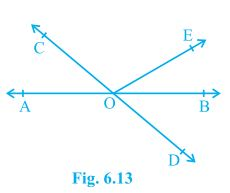Given,
∠AOC + ∠BOE = 70° and ∠BOD = 40°
A/q,
∠AOC + ∠BOE +∠COE = 180° (Forms a straight line)
⇒ 70° +∠COE = 180°
⇒ ∠COE = 110°
also,
∠COE +∠BOD + ∠BOE = 180° (Forms a straight line)
⇒ 110° +40° + ∠BOE = 180°
⇒ 150° + ∠BOE = 180°
⇒ ∠BOE = 30°

Page No: 97

2. In Fig. 6.14, lines XY and MN intersect at O. If ∠POY = 90° and a : b = 2 : 3, find c.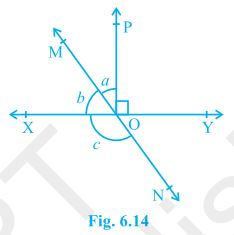Given,
∠POY = 90° and a : b = 2 : 3
A/q,
∠POY + a + b = 180°
⇒ 90° + a + b = 180°
⇒ a + b = 90°
Let a be 2x then will be 3x
2x + 3x = 90°
⇒ 5x = 90°
⇒ x = 18°
∴ a = 2×18° = 36°
and b = 3×18° = 54°
also,
b + c = 180° (Linear Pair)
⇒ 54° + c = 180°
⇒ c = 126°

3. In Fig. 6.15, ∠PQR = ∠PRQ, then prove that ∠PQS = ∠PRT.Given,
∠PQR = ∠PRQ
To prove,
∠PQS = ∠PRT
A/q,
∠PQR +∠PQS = 180° (Linear Pair)
⇒ ∠PQS = 180° - ∠PQR --- (i)
also,
∠PRQ +∠PRT = 180° (Linear Pair)
⇒ ∠PRT = 180° - ∠PRQ
⇒ ∠PRQ = 180° - ∠PQR --- (ii) (∠PQR = ∠PRQ)
From (i) and (ii)
∠PQS = ∠PRT = 180° - ∠PQR
Therefore,  ∠PQS = ∠PRT

4. In Fig. 6.16, if x + y = w + z, then prove that AOB is a line.Given,
x + y = w + z
To Prove,
AOB is a line or x + y = 180° (linear pair.)
A/q,
x + y + w + z = 360° (Angles around a point.)
⇒ (x + y) +  (w + z) = 360°
⇒ (x + y) +  (x + y) = 360° (Given x + y = w + z)
⇒ 2(x + y) = 360°
⇒ (x + y) = 180°
Hence, x + y makes a linear pair. Therefore, AOB is a staright line.

5. In Fig. 6.17, POQ is a line. Ray OR is perpendicular to line PQ. OS is another ray lying between rays OP and OR. Prove that ∠ROS = 1/2(∠QOS – ∠POS).Given,
OR is perpendicular to line PQ
To prove,
∠ROS = 1/2(∠QOS – ∠POS)
A/q,
∠POR = ∠ROQ = 90° (Perpendicular)
∠QOS = ∠ROQ + ∠ROS = 90° + ∠ROS --- (i)
∠POS = ∠POR - ∠ROS = 90° - ∠ROS --- (ii)
Subtracting (ii) from (i)
∠QOS - ∠POS = 90° + ∠ROS - (90° - ∠ROS)
⇒ ∠QOS - ∠POS = 90° + ∠ROS - 90° + ∠ROS
⇒ ∠QOS - ∠POS = 2∠ROS
⇒ ∠ROS = 1/2(∠QOS – ∠POS)
Hence, Proved.

6. It is given that ∠XYZ = 64° and XY is produced to point P. Draw a figure from the given information. If ray YQ bisects ∠ZYP, find ∠XYQ and reflex ∠QYP.

Given,
∠XYZ = 64°
YQ bisects ∠ZYP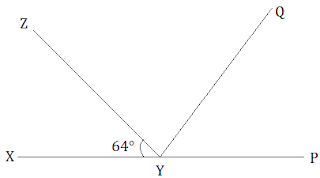∠XYZ +∠ZYP = 180° (Linear Pair)
⇒ 64° +∠ZYP = 180°
⇒ ∠ZYP = 116°
also, ∠ZYP = ∠ZYQ + ∠QYP
∠ZYQ = ∠QYP (YQ bisects ∠ZYP)
⇒ ∠ZYP = 2∠ZYQ
⇒ 2∠ZYQ = 116°
⇒ ∠ZYQ = 58° = ∠QYP
Now,
∠XYQ = ∠XYZ + ∠ZYQ
⇒ ∠XYQ = 64° + 58°
⇒ ∠XYQ = 122°
also,
reflex ∠QYP = 180° + ∠XYQ
∠QYP = 180° + 122°
⇒ ∠QYP = 302°

Page No: 103

Exercise 6.2

1. In Fig. 6.28, find the values of x and y and then show that AB || CD.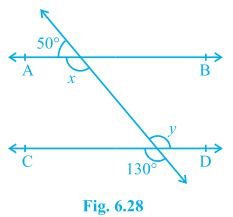x + 50° = 180° (Linear pair)
⇒ x = 130°
also,
y = 130° (Vertically opposite)
Now,
x = y = 130° (Alternate interior angles)
Alternate interior angles are equal.
Therefore, AB || CD.

Page No: 104

2. In Fig. 6.29, if AB || CD, CD || EF and y : z = 3 : 7, find x.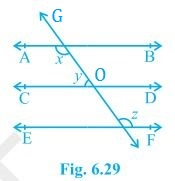Given,
AB || CD and CD || EF
y : z = 3 : 7
Now,
x + y = 180° (Angles on the same side of transversal.)
also,
∠O = z (Corresponding angles)
and, y + ∠O = 180° (Linear pair)
⇒ y + z = 180°
A/q,
y = 3w and z = 7w
3w + 7w = 180°
⇒ 10 w = 180°
⇒ w = 18°
∴ y = 3×18° = 54°
and, z = 7×18° = 126°
Now,
x + y = 180°
⇒ x + 54° = 180°
⇒ x = 126°

3. In Fig. 6.30, if AB || CD, EF ⊥ CD and ∠GED = 126°, find ∠AGE, ∠GEF and ∠FGE.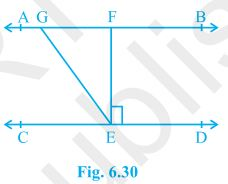Given,
AB || CD
EF ⊥ CD
∠GED = 126°
A/q,
∠FED = 90° (EF ⊥ CD)
Now,
∠AGE = ∠GED (Since, AB || CD and GE is transversal. Alternate interior angles.)
∴ ∠AGE = 126°
Also, ∠GEF = ∠GED - ∠FED
⇒ ∠GEF = 126° - 90°
⇒ ∠GEF = 36°
Now,
∠FGE +∠AGE = 180° (Linear pair)
⇒ ∠FGE = 180° - 126°
⇒ ∠FGE = 54°

4. In Fig. 6.31, if PQ || ST, ∠PQR = 110° and ∠RST = 130°, find ∠QRS.
[Hint : Draw a line parallel to ST through point R.]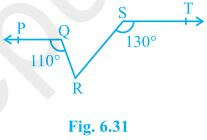Given,
PQ || ST, ∠PQR = 110° and ∠RST = 130°
Construction,
A line XY parallel to PQ and ST is drawn.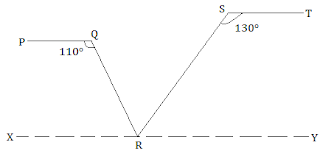∠PQR + ∠QRX = 180° (Angles on the same side of transversal.)
⇒ 110° + ∠QRX = 180°
⇒ ∠QRX = 70°
Also,
∠RST + ∠SRY = 180° (Angles on the same side of transversal.)
⇒ 130° + ∠SRY = 180°
⇒ ∠SRY = 50°
Now,
∠QRX +∠SRY + ∠QRS = 180°
⇒ 70° + 50° + ∠QRS = 180°
⇒ ∠QRS = 60°

5. In Fig. 6.32, if AB || CD, ∠APQ = 50° and ∠PRD = 127°, find x and y.Given,
AB || CD, ∠APQ = 50° and ∠PRD = 127°
A/q,
x = 50° (Alternate interior angles.)
∠PRD + ∠RPB = 180° (Angles on the same side of transversal.)
⇒ 127° + ∠RPB = 180°
⇒ ∠RPB = 53°
Now,
y + 50° + ∠RPB = 180° (AB is a straight line.)
⇒ y + 50° + 53° = 180°
⇒ y + 103° = 180°
⇒ y = 77°

6. In Fig. 6.33, PQ and RS are two mirrors placed parallel to each other. An incident ray AB strikes the mirror PQ at B, the reflected ray moves along the path BC and strikes the mirror RS at C and again reflects back along CD. Prove that AB || CD.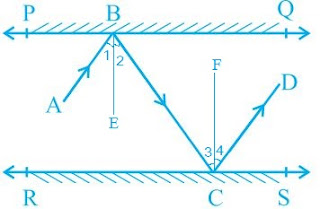Let us draw BE ⟂ PQ and CF ⟂ RS.
As PQ || RS
So, BE || CF
By laws of reflection we know that,
Angle of incidence = Angle of reflection
Thus, ∠1 = ∠2 and ∠3 = ∠4  --- (i)
also, ∠2 = ∠3     (alternate interior angles because BE || CF and a transversal line BC cuts them at B and C)    --- (ii)
From (i) and (ii),
∠1 + ∠2 = ∠3 + ∠4
⇒ ∠ABC = ∠DCB
⇒ AB || CD      (alternate interior angles are equal)

Page No: 107

Exercise 6.3

1. In Fig. 6.39, sides QP and RQ of ΔPQR are produced to points S and T respectively. If ∠SPR = 135° and ∠PQT = 110°, find ∠PRQ.Given,
∠SPR = 135° and ∠PQT = 110°
A/q,
∠SPR +∠QPR = 180° (SQ is a straight line.)
⇒ 135° +∠QPR = 180°
⇒ ∠QPR = 45°
also,
∠PQT +∠PQR = 180° (TR is a straight line.)
⇒ 110° +∠PQR = 180°
⇒ ∠PQR = 70°
Now,
∠PQR +∠QPR + ∠PRQ = 180° (Sum of the interior angles of the triangle.)
⇒ 70° + 45° + ∠PRQ = 180°
⇒ 115° + ∠PRQ = 180°
⇒ ∠PRQ = 65°

2. In Fig. 6.40, ∠X = 62°, ∠XYZ = 54°. If YO and ZO are the bisectors of ∠XYZ and ∠XZY respectively of Δ XYZ, find ∠OZY and ∠YOZ.Given,
∠X = 62°, ∠XYZ = 54°
YO and ZO are the bisectors of ∠XYZ and ∠XZY respectively.
A/q,
∠X +∠XYZ + ∠XZY = 180° (Sum of the interior angles of the triangle.)
⇒ 62° + 54° + ∠XZY = 180°
⇒ 116° + ∠XZY = 180°
⇒ ∠XZY = 64°
Now,
∠OZY = 1/2∠XZY (ZO is the bisector.)
⇒ ∠OZY = 32°
also,
∠OYZ = 1/2∠XYZ (YO is the bisector.)
⇒ ∠OYZ = 27°
Now,
∠OZY +∠OYZ + ∠O = 180° (Sum of the interior angles of the triangle.)
⇒ 32° + 27° + ∠O = 180°
⇒ 59° + ∠O = 180°
⇒ ∠O = 121°

3. In Fig. 6.41, if AB || DE, ∠BAC = 35° and ∠CDE = 53°, find ∠DCE.Given,
AB || DE, ∠BAC = 35° and ∠ CDE = 53°
A/q,
∠BAC = ∠CED (Alternate interior angles.)
∴ ∠CED = 35°
Now,
∠DCE +∠CED + ∠CDE = 180° (Sum of the interior angles of the triangle.)
⇒ ∠DCE + 35° + 53° = 180°
⇒ ∠DCE + 88° = 180°
⇒ ∠DCE = 92°

4. In Fig. 6.42, if lines PQ and RS intersect at point T, such that ∠PRT = 40°, ∠RPT = 95° and ∠TSQ = 75°, find ∠SQT.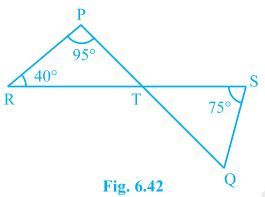Given,
∠PRT = 40°, ∠RPT = 95° and ∠TSQ = 75°
A/q,
∠PRT +∠RPT + ∠PTR = 180° (Sum of the interior angles of the triangle.)
⇒ 40° + 95° + ∠PTR = 180°
⇒ 40° + 95° + ∠PTR = 180°
⇒ 135° + ∠PTR = 180°
⇒ ∠PTR = 45°
∠PTR = ∠STQ = 45° (Vertically opposite angles.)
Now,
∠TSQ +∠PTR + ∠SQT = 180° (Sum of the interior angles of the triangle.)
⇒ 75° + 45° + ∠SQT = 180°
⇒ 120° + ∠SQT = 180°
⇒ ∠SQT = 60°

Page No: 108

5. In Fig. 6.43, if PQ ⊥ PS, PQ || SR, ∠SQR = 28° and ∠QRT = 65°, then find the values of x and y.Given,
PQ ⊥ PS, PQ || SR, ∠SQR = 28° and ∠QRT = 65°
A/q,
x +∠SQR = ∠QRT (Alternate angles  as QR is transveersal.)
⇒ x + 28° = 65°
⇒ x = 37°
also,
∠QSR = x
⇒ ∠QSR = 37°
also,
∠QRS +∠QRT = 180° (Linea pair)
⇒ ∠QRS + 65° = 180°
⇒ ∠QRS = 115°
Now,
∠P + ∠Q+ ∠R +∠S = 360° (Sum of the angles in a quadrilateral.)
⇒ 90° + 65° + 115° + ∠S = 360°
⇒ 270° + y + ∠QSR = 360°
⇒ 270° + y + 37° = 360°
⇒ 307° + y = 360°
⇒ y = 53°

6. In Fig. 6.44, the side QR of ΔPQR is produced to a point S. If the bisectors of ∠PQR and ∠PRS meet at point T, then prove that ∠QTR = 1/2∠QPR.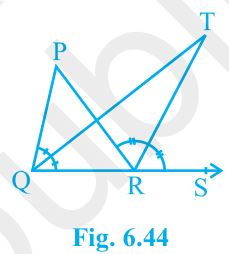Given,
Bisectors of ∠PQR and ∠PRS meet at point T.
To prove,
∠QTR = 1/2∠QPR.
Proof,
∠TRS = ∠TQR +∠QTR (Exterior angle of a triangle equals to the sum of the two interior angles.)
⇒ ∠QTR = ∠TRS - ∠TQR --- (i)
also,
∠SRP = ∠QPR + ∠PQR
⇒ 2∠TRS = ∠QPR + 2∠TQR
⇒ ∠QPR =  2∠TRS - 2∠TQR
⇒ 1/2∠QPR =  ∠TRS - ∠TQR --- (ii)
Equating (i) and (ii)
∠QTR - ∠TQR = 1/2∠QPR
Hence proved.

## NCERT Solutions for Class 9 Maths Chapter 6 Lines and Angles

Chapter 6 Lines and Angles is a great chapter which must be in your strategy to improve your marks in geometry section. You will learn variety of new terms and definitions which are going to help in solving questions.

• Basic Terms and Definitions: A part of a line with two end points is called a line-segment and a part of a line with one end point is called a ray. If three or more points lie on the same line, they are called collinear points otherwise they are called non-collinear points. An angle is formed when two rays originate from the same end point. The rays making an angle are called the arms of the angle and the end point is called the vertex of the angle.

An acute angle measures between 0° and 90°, whereas a right angle is exactly equal to 90°. An angle greater than 90° but less than 180° is called an obtuse angle. A straight angle is equal to 180°. An angle which is greater than 180° but less than 360° is called a reflex angle. Two angles whose sum is 90° are called complementary angles, and two angles whose sum is 180° are called supplementary angles. Two angles are adjacent, if they have a common vertex, a common arm and their non-common arms are on different sides of the common arm.

• Intersecting Lines and Non-intersecting Lines: If two lines intersect each other, then the vertically opposite angles are equal.

• Pairs of Angles: There is a very important theorem is given in this section. On the basis of which you have to solve questions given in the exercise 6.1 If two lines intersect each other, then the vertically opposite angles are equal.

• Parallel Lines and a Transversal: There are four theorems given in this section which is to going to help you in solving questions effectively.
(i) If a transversal intersects two parallel lines, then each pair of alternate interior angles is equal.
(ii) If a transversal intersects two lines such that a pair of alternate interior angles is equal, then the two lines are parallel.
(iii) If a transversal intersects two parallel lines, then each pair of interior angles on the same side of the transversal is supplementary.
(iv) If a transversal intersects two lines such that a pair of interior angles on the same side of the transversal is supplementary, then the two lines are parallel.

• Lines Parallel to the Same Line: Lines which are parallel to the same line are parallel to each other.

• Angle Sum Property of a Triangle: (i) The sum of the angles of a triangle is 180º. (ii) If a side of a triangle is produced, then the exterior angle so formed is equal to the sum of the two interior opposite angles.

Three exercises are given in Chapter 6 Lines and Angles NCERT Solutions which will improve your knowledge of Geometry. Every students must try to solve each questions given in the exercise that is why we have also provided exercise wise solutions of every problem that you can find below.

Studyrankers experts at every step, they have tried to prepare these Class 9 Maths NCERT Solutions in such a way that you can easily understand even the most difficult problems. You can always clear related to this chapter just by visiting this page.

### NCERT Solutions for Class 9 Maths Chapters:

 Chapter 1 Number Systems Chapter 2 Polynomials Chapter 3 Coordinate Geometry Chapter 4 Linear Equations in Two Variables Chapter 5 Introduction to Euclid’s Geometry Chapter 7 Triangles Chapter 8 Quadrilaterals Chapter 9 Areas of Parallelograms and Triangles Chapter 10 Circles Chapter 11 Constructions Chapter 12 Heron's Formula Chapter 13 Surface Areas And Volumes Chapter 14 Statistics Chapter 15 Probability

FAQ on Chapter 6 Lines and Angles

#### How many exercises in Chapter 6 Lines and Angles?

There are only three exercise in Chapter 6 Lines and Angles NCERT Solutions which are also important for competitive exams and higher grades. We have detailed every step through which one can always clear their doubts.

#### If the supplement of an angle is 4 times of its complement, find the angle.

Let the required angle be x
∴ (180°- x) = 4(90° - x)
⇒ x = 60°

#### What is the measure of an angle whose measure is 32° less than its supplement?

Let the required angle be x
∴ x = (180°- x) - 32°
⇒ x = 74°

#### Angles ∠P and 100° form a linear pair. What is the measure of ∠P?

Since, the sum of the angles of a linear pair equal to 180°.
∴ ∠ P + 100° = 180°
⇒ ∠ P = 180° - 100 = 80°.